# Precalculus : Solve Systems of Linear Equations

## Example Questions

← Previous 1 2 Next →

### Example Question #11 : Inequalities And Linear Programming

Find the point of intersection by using Gaussian elimination: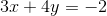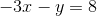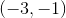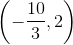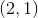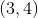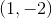Explanation:

To solve this, let's first try to eliminate x. We can do this by adding the two equations: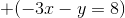-------------------------------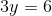Which implies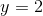We can now solve for x by plugging 2 in for y in either equation.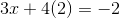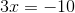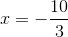### Example Question #11 : Inequalities And Linear Programming

Solve the following system of equations: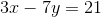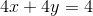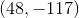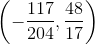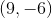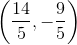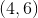Explanation:

Let's solve this equation by eliminating the variable x by adding a multiple of the second equation to the first.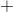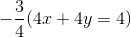---------------------------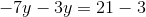Now let's combine those y values and solve for y.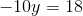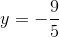Now all we have to do is plug that in for y in either original equation to solve for x.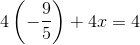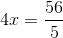Thus this yields the intersection point### Example Question #11 : Solve Systems Of Linear Equations

Solve the following system of equations for the intersection point in space: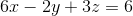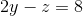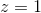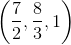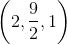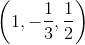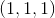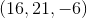Explanation:

Because one of the variables, z, has already been isolated, let's use the substitution method to solve this system of equations. We know z = 1, so let's plug that into the middle equation to solve for y: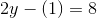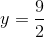Now that we have found y, let's solve for x by plugging both y and z into the top equation: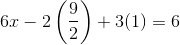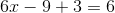Thus we have found that the point of intersection would be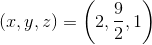← Previous 1 2 Next →

### All Precalculus Resources